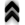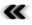### 3.3 Example of Generic Programming

To demonstrate the facilities and opportunities of the described methods a short example calculating the value of a determinant of a matrix is presented. While relatively simple in terms of mathematical sophistication, it provides the opportunity to show the procedures and methods of generic programming in the C++ programming language , which diﬀer quite signiﬁcantly from those usually prevalent in C++ run time expressions.

The selection of the C++ programming language for use in examples was made according to the criteria provided in Section 3.1.5, starting with the fact that C++ is a multi paradigmatic programming language, since it supports the following programming paradigms :

• Structured imperative – as a direct derivative of the C language
• Object-oriented – it has direct support for inheritance and virtual functions
• Functional programming – using libraries such as Boost Phoenix 
• Generic programming – using the meta programming facilities

The currently unique combination of features present in C++, makes it highly suitable for deployment in the ﬁeld of scientiﬁc computing. Furthermore, not only is a mature tool chain for C++ available, but generic programming has been a part of the C++ programming language in the form of the STL  form the initial standardization. The underlying design patterns have since been examined  and elaborated [38 ]. Additionally, the mechanisms directly supporting generic programming in C++ have been the subject of investigation  as well as the performance penalties encountered due to abstractions in generic programming . With the advent of multi-core CPUs, the issue of parallelization has increased in importance and has been shown to be compatible with generic programming in C++ . Finally, generic programming in C++ has already been successfully applied to scientiﬁc computing .

Concepts for scientiﬁc computing have already been impressively demonstrated as applicable for topological frameworks especially for the use in numerical schemes to solve partial diﬀerential equations due to physical problems . Building on such a ﬁrm base and since examples are already broadly available, this thesis attempts to investigate the interaction and interrelation of the realm of topology and geometry. A particular contribution of this work deals with the physical phenomena between both classical and quantum descriptions using integral descriptions, which are connected to the geometric aspects found in theory. While this work shares fundamental components of topology and their implementation, it is the abstract geometrical requirements which are a driving force for the investigation and application of programming concepts. As source code is much more easily constructed than ideas and thought patterns, it is the attitude with which to approach and solve problems which is of primary concern and which has been further developed.

Since the implementation makes use of both, run time and compile time structures, special consideration of elements crossing the border between these two worlds is required. To make eﬃcient use of the facilities provided by meta programming, generic programming methods necessarily need to cross this border, as every of these points of evaluation embosses their respective advantages and disadvantages on the executable. Only their compound use can converge to optimal use.

#### 3.3.1 Mathematical Description

Determinants have several applications from orientation tests to eigenvalue problems. A very simple algorithm to calculate the value of a determinant, is to apply Laplace expansion to the determinant. While it is not the most eﬃcient algorithm, it is well suited to demonstrate the use of a generic C++ program incorporating template meta programming techniques, due to its recursive nature. For completeness the algorithm is outlined shortly before showing an implementation.

Given a matrix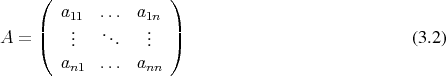its determinant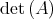can be calculated by breaking it down into a series of smaller determinants. To this end a columnis chosen to arrive at the formula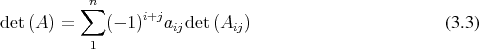The matrices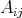are minors to the original matrix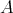, meaning that the rows and columns indicated by the indices are omitted to obtain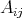. This procedure can be performed recursively until the determinant expressions are already known, such as is the case for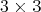or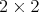matrices or even the trivial case of a single value. The ﬁnal expression is then of a form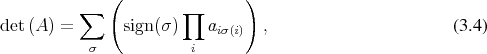where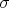is a permutation and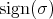the sign of the permutation. The recursive nature of the construction of this sum of products makes this algorithm well suited for implementation using the functional style required by template meta programming.

It favours the implementation of an algorithm, how the correct access patterns into the data are to be constructed and how to combine this data. Thus while patterns of access will be completely available at compile time, the actual values, which will be manipulated may be supplied at run time. This distinction oﬀers the compiler the complete set of information of memory accesses and it can therefore do its best to optimize them. However it should not go unnoted that the optimizer may be overwhelmed by the amount of data connected to the calculation as the size of matrices increases. While the consequences depend on the particular optimizer in use by the compiler, a sub optimal solution is the most likely result, with an abnormal termination being an extreme, but not impossible case.

The structural, purely compile time parts shall be given ﬁrst. It constructs sequences of types, which encode the required operations. These operations are then carried out using a run time adaptor. This results in a clear and complete separation of the algorithmic structure created at compile time and the data structure used to store actual values. The compile time structure in this case is thus equivalent to a computer program written by a programmer by hand, only that it is constructed algorithmically from the structural rules provided by the mathematical description. The use of the algorithm requires the binding of the compile time prescription to run time values. Since only when combining a run time matrix accessor with the compile time structure an algorithm for the calculation of the value of the determinant is obtained. Otherwise the compile time structure merely implements a means of determining permutations as is evident from Equation 3.4. This fact is also an indication that the description here is for demonstration purposes of a venue for generic programing, instead of considering it for high performance determinant calculations. In this regard it would be much more ﬁtting to use an implementation following Gaussian elimination, LU or QR factorization, which, while still costly, have far less computational complexity than the number of permutations.

#### 3.3.2 Compile Time Structure

It should be recalled that C++ meta programs are completely stateless, making recursion the only option to express repetitions. In the following the mpl name space indicator shall be short for boost::mpl which indicates the Boost MPL library .

Examining the terms involved in Equation 3.3 and Equation 3.4 provides the guidelines for the deﬁnition of the compile time structure. The recursion to sub determinants is a means of construction all the required permutations required for the calculation of the determinant. Thus the necessary tasks may be broken down into the following steps

• Determine a mechanism to encode a single element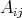.
• Choose a representation for the multiplication of elements.
• Incorporate the sign associated with a permutation.
• Set an encoding for the sequence of additions.

A short outline of each of these steps is provided before the meta programs for the generation and manipulation are detailed.

Access to any of the elements of the matrix data set is determined by the pair of indices. To encode this in a compile time structure, the indices are encoded into integral types such as mpl::long_ and encapsulated into a mpl::pair. Thus, a matrix element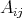shall be represented by:

Listing 3.1: A pair of indices encoded into an mpl::pair.
typedef mpl::long_<i> pos_i;
typedef mpl::long_<j> pos_j;

typedef mpl::pair<pos_i,pos_j>::type matrix_element_type;

This encoding concerns itself purely with the position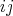, not with the value stored at the indicated position.

The multiplication of thusly encoded elements is accomplished utilizing a simple type sequence, the mpl::vector.

Listing 3.2: A sequence of pairs representing elements for multiplications.
typedef mpl::pair<pos_i1,pos_j1>::type matrix_element_type_1;
typedef mpl::pair<pos_i2,pos_j2>::type matrix_element_type_2;
typedef mpl::vector<matrix_element_type_1,
matrix_element_type_2>::type mult_sequence;

In order to bond a sign, which is encoded as a integral constant, to the multiplication sequence an mpl::pair is used:

Listing 3.3: A sequence of pairs representing elements for multiplications.
typedef mpl::pair<sign_type,
mult_sequence>::type mult_signed_sequence;

The resulting sequences are then collected within an mpl::vector which encodes all the necessary operations.

Listing 3.4: A sequence of pairs representing elements for multiplications.
typedef mpl::vector<mult_signed_sequence_1,
mult_signed_sequence_2
>::type determinant_structure_type;

Having outlined the means of representation, the means with which to generate these representations is sill missing and is provided in the following.

#### 3.3.3 Compile Time Meta Program

The top level of the compile time program takes the form of a simple struct containing the invocation of the recursive meta program components with the natural order of the indices. It accepts a sole input parameter indicating the size of the data set.

Listing 3.5: Top level of the determinant meta program.
template<long size>
struct determinant_struct_sequence
{
typedef typename mpl::range_c<long,0,size>::type initial_map;
typedef typename recursive_determinant<initial_map, initial_map,
size>::type type;
};

The recursive part is given in the next piece of code. Particularly it is the specialization for two-dimensional data sets, which ends the recursion. It uses the well known prescription to calculate the determinant in this case. To this end the correct mappings are extracted into the types x0, x1, y0 and y1. The obtained indices are then paired into an mpl::pair which speciﬁes the access to a single point within the data set.

The pairs are enclosed into compile time containers, mpl::vectors, which encode the multiplication of the pairs it contains. This has the beneﬁt of making the multiplications extensible in a simple fashion. The two possible conﬁgurations of the two-dimensional case are represented by the types first and second for the term corresponding to the main diagonal and the oﬀ diagonal terms respectively.

Finally the two types corresponding to multiplication are packaged into another pair, which now links the sign with which the expression is to be evaluated to the multiplications, and assembled into another compile time container which is used to store the additive terms. It is this compile time container which is returned by the meta function.

Listing 3.6: A two-dimensional determinant serves as the end of the recursion of the determinant meta program.
template<typename MappingTypeX, typename MappingTypeY>
struct recursive_determinant<MappingTypeX, MappingTypeY, 2>
{
typedef typename mpl::at_c<MappingTypeX,0>::type x0;
typedef typename mpl::at_c<MappingTypeX,1>::type x1;

typedef typename mpl::at_c<MappingTypeY,0>::type y0;
typedef typename mpl::at_c<MappingTypeY,1>::type y1;

typedef typename mpl::vector<typename mpl::pair<x0,y0>::type,
typename mpl::pair<x1,y1>::type
>::type first;

typedef typename mpl::vector<typename mpl::pair<x1,y0>::type,
typename mpl::pair<x0,y1>::type
>::type second;

typedef typename mpl::vector<
typename mpl::pair<typename mpl::long_<1>::type,
first>::type,
typename mpl::pair<typename mpl::long_<1>::type,
second>::type>::type type;
};

Having established how to calculate a two-dimensional determinant as the end of a recursion, the general deﬁnition of the recursive component is discussed next. The parameters are the same as in the two-dimensional case, with the exception of leaving the size unspecialized.

The implementation then proceeds by omitting an element from one of the index sequences. The return type is constructed by folding a parametrized functor over the sequence of indices, whose consecutive results are gathered within a compile time container. The types generated by the repeated functor invocations encode the terms which need to be summed for the calculation of the value of the determinant.

Listing 3.7: Main recursion meta algorithm.
template<typename MappingTypeX, typename MappingTypeY,
long matrix_size>
struct recursive_determinant
{
typedef typename omit_view_c<MappingTypeY,
0>::type remappedY;

typedef typename mpl::range_c<long
0, matrix_size>::type steps;

typedef typename mpl::fold<
steps,
mpl::vector<>
recurse<MappingTypeX, remappedY,
typename mpl::at_c<MappingTypeY,0>::type>
>::type type;
};

The just described section of code was referred to as being recursive, however no obviously recursive call is directly discernible. The truly recursive nature is revealed by examining the employed functor in more detail. The functor follows Boost MPL semantics for functors by containing a nested struct named apply. The additional information of the outside mappings along with the currently discarded index are supplied directly to the recurse template, while the nested apply is fed with arguments accumulating previous evaluations as well as the current evaluation item during the fold.

It is the functor’s task to construct determinants for the minors within the apply struct. To this end it omits an element from the so far unaltered index sequence, thus creating an expression corresponding to a minor of the given matrix, which in turn is passed to the recursive determinant meta function recursive_determinant. The element corresponding to the omitted row and column for the construction of the minor is again encoded in a pair along with the appropriate sign, which is also fully determined at compile time using a utility meta function sign. This element is multiplied with the result from the evaluation of the minor’s determinant, as indicated in Equation 3.3. This multiplication is accomplished by appropriately inserting the signed local element into the compile time container generated for the sub determinant. The ﬁnal result is obtained by concatenating the current result with the results obtained from previous calls using the mpl::joint_view construct.

Listing 3.8: Functor used to obtain the return type of the recursive determinant meta function.
template<typename MappingTypeX, typename MappingTypeY,
typename LostType>
struct recurse
{
template<typename StateType, typename c>
struct apply
{
static const size_t length =
mpl::size<MappingTypeX>::type::value;

typedef typename omit_view<MappingTypeX,c>::type
reduced_mapping;
typedef typename recursive_determinant<reduced_mapping,
MappingTypeY,
length1>::type
sub_determinant_type;

typedef typename mpl::at<MappingTypeX,c>::type current_index;

typedef typename mpl::pair<current_index,
LostType>::type
local_element_type;
typedef typename mpl::pair<
typename mpl::long_<sign<c::value>::value>::type,
local_element_type>::type signed_local_element_type;

typedef typename append_to_subsequences<sub_determinant_type,
signed_local_element_type>::type
sub_multiplied_type;

typedef typename mpl::joint_view<StateType,
sub_multiplied_type>::type
type;
}
};

The next snippet of code shows a simple calculation at compile time, as used to determine the sign. Note that only simple operators and static const integer types are available.

Listing 3.9: Utility meta function for sign calculation.
template<long exponent>
struct sign
{
static const long value = (exponent % 2) ? 1 : 1;
};

The next piece of code shows how intermediate results are concatenated to appropriately encode the desired mathematical operations. The procedure is again split into two components. The top level meta function being:

Listing 3.10: Utility meta function used to correctly combine intermediate result.
template<typename SequenceType, typename ItemType>
struct append_to_subsequences
{
typedef typename mpl::fold<
SequenceType,
mpl::vector<>
push_back<mpl::_1,
signed_inserter<mpl::_2,ItemType> >
>::type type;
};

It should not go unnoted that the push_back here is a meta function working on the compile time container mpl::vector in the same fashion as the run time equivalent would on a std::vector. However, where the run time function simply modiﬁes an existing container, the compile time version generates a completely new sequence, due to the immutable nature of C++ meta programming. The mpl::_1 and mpl::_2 are named to resemble their run time lambda expressions.

A further utility meta function signed_inserter is employed to transform the elements of the input sequence before pushing them into the result sequence, in order to match the chosen logical encoding.

Listing 3.11: Generic algorithm for calculating determinants compile time meta program.
template<typename SignedSequType, typename ItemType>
struct signed_inserter
{
typedef typename SignedSequType::first  initial_sign_type;
typedef typename SignedSequType::second coefficients_type;

typedef typename ItemType::second item_type;

typedef typename mpl::times<initial_sign_type,
sign_type;

typedef typename mpl::push_back<coefficients_type,
item_type>::type
extended_sequence_type;
typedef typename mpl::pair<sign_type,
extended_sequence_type>::type type;
};

First the input elements, which are expected to be pairs, are unpacked. Then the resulting sign is calculated by using the mpl::times meta function, which works on integral constant types such as mpl::int_, on the extracted individual signs. The element to be added is pushed into the coeﬃcient sequence. It should again be noted that this does not mutate the original sequence in any way, but rather results in a completely new sequence, which contains the original types along with the pushed type. The extended sequence is then repacked together with the calculated sign type to resulting type of this meta function.

All the meta functions so far are suﬃcient to generate a structure of types, which encodes all the operations associated with a determinant, by providing all the required permutations at compile time. This structural part is in fact completely independent from the task of computing determinants and can be freely reused beyond the ﬁeld of matrices and matrix data types. Since this part of the algorithm is not tied to the domain of matrices, this implementation, which follows the mathematical description very closely, is consequently completely independent of the matrix data type for which a determinant is to be calculated. It therefore surpasses any plain attempt to arrive at data type independence using a simpler template approach, which attempts to simply make a generic implementation from a procedural implementation by converting it to template functions, since that will implicitly introduce the matrix data type. It is this implicit nature which degrades the level of generality.

The components shown this far solely belong to the compile time regime. In order to enable the evaluation of determinants whose values are determined at run time, additional facilities are required which bridge the run time / compile time border.

The ﬁrst component of the run time adaption deals with the evaluation of multiplications. The following unary function object is a key component of this endeavour:

Listing 3.12: Function object for the evaluation of the multiplicative sequences.
template<typename MatrixAccessorType, typename NumericType>
struct multiplication_sequence_eval
{
const MatrixAccessorType& matrix;
NumericType& return_value;
multiplication_sequence_eval(const MatrixAccessorType& matrix,
NumericType& return_value) :
matrix(matrix), return_value(return_value) {}

template< typename U >
inline void operator()(U x)
{
typedef typename U::first  first;
typedef typename U::second second;

return_value = matrix(first::value, second::value);
}
};

It is parametrized on the access mechanism to the data contained within the matrix as well as the numeric type indicating the type of the result of the computations. Matrix access is held by constant reference within the object, thus being able to access the data in place in a safe manner. The return value is also used by reference and hence also has to be supplied at construction time.

Evaluation of the result of the multiplicative sequence takes place in the templated operator(). The parameter it formally takes is merely a dummy whose value is never actually used. It is used solely for type deduction. The indices for access to the matrix are extracted from the supplied type and fed into the matrix access mechanism. In this fashion consecutive evaluations of the operator along a type sequence enable to evaluation of the multiplication of the matrix elements indicated by the indices. A fact which might escape attention due to the compactness of the speciﬁcation using the template mechanism is that each individual type within the type sequence will spawn its own implementation of operator() specialized to appropriately.

Another unary function object determinant_structure_eval is used to not only initiate the iteration required by the multiplication_sequence_eval function object, but also to aggregate the individual results of multiplication.

Listing 3.13: Function object for the aggregation of multiplicative terms.
template<typename MatrixAccessorType, typename NumericType>
struct determinant_structure_eval
{
const MatrixAccessorType& matrix;
NumericType& result;

determinant_structure_eval(const MatrixAccessorType& matrix,
NumericType& result) :
matrix(matrix), result(result) {}

template<typename SignedSequenceType>
inline void operator()(SignedSequenceType X)
{
typedef typename SignedSequenceType::first  sign_type;
typedef typename SignedSequenceType::second sequence_type;

NumericType local_result(1);

mpl::for_each<sequence_type>
(multiplication_sequence_eval<MatrixAccessorType,
NumericType>(matrix,
local_result));

result += sign_type::value  local_result;

}
};

The structure of the function object follows exactly the same pattern as in the previous case. The diﬀerence lies solely in the computations within operator(). The sequence of multiplications is extracted and evaluated using the MPL’s sole run time algorithm, mpl::for_each, which traverses the type sequence and invokes a supplied unary function object. By using the multiplication_sequence_eval function object, the terms indicated in the type sequence are multiplied. Finally, the sign of the current term, which has been extracted from the input data type, is applied in the ﬁnal addition.

Now that the components have been deﬁned it is possible to deﬁne a top level interface for the calculation of a determinant. Casting it into the form of a function object:

Listing 3.14: Top level interface.
template<typename DeterminantStructure>
struct determinant_interface
{
template<typename MatrixAccessorType, typename NumericT>
inline void operator()(const MatrixAccessorType& matrix_access,
NumericT& result)
{
result = NumericT(0);
mpl::for_each<DeterminantStructure>
determinant_structure_eval<MatrixAccessorType,
NumericT>(matrix_access,result) );
}
};

Here the determinant structure has to be speciﬁed explicitly during type creation, while the MatrixAccessorType and NumericType can be derived automatically from the arguments of the operator(). This allows for the reuse of the function object for matrices of the same size, and hence of the same structure, but diﬀerent matrix types or diﬀerent numerical requirements.

Reexamining the provided run time implementations allows to assess the requirements placed on the matrix and numeric data types. The matrix data type’s requirement is determined by its use in Listing 3.12. It is required that an operator() is available with which to access the data within the matrix, when supplied with two integer type arguments. No further restrictions, such as memory layout, apply in the given implementation for matrices. In fact anything complying with the required interface will be considered a valid matrix with respect to evaluation.

The requirements enforced on the numeric data type, beside being capable of the basic numeric operations of addition and multiplication, are on the one hand connected to a convertibility/assignability of the result of accessing the matrix to the numeric type, as seen in Listing 3.12. Additionally the numeric type needs to be constructable as done in Listing 3.13 and Listing 3.14.

To complete the given example, the application of the compile time determinant structure and the given run time evaluations is presented using a simple matrix constructed from std::vector. Since this representation does not provide the required access mechanism, a thin wrapper is required. It is presented in Listing 3.15 and shows how access is remapped to the inherent mechanisms.

Listing 3.15: Application of the compile time determinant.
template<typename MatrixT>
struct vector_vector_like_matrix
{
const MatrixT& matrix;
vector_vector_like_matrix(const MatrixT& mx):matrix(mx){}

double operator()(long x, long y) const
{
return matrix[x][y];
}
};

Equipped with the wrapper, the ﬁnal deployment is illustrated in Listing 3.16. First, the compile time program is evoked, resulting in a data type encoding the instructions to be processed. It requires no information about the run time values of the matrix but takes only the size of the matrix as input. Next, the matrix type is deﬁned and instantiated and this instance bound to the wrapper shown in Listing 3.15. Omitting the insertion of values into the matrix the snippet of code proceeds to provide a variable to contain the result and ﬁnally applies the compile time algorithm to the matrix via the matrix_access wrapper, thus completing the demonstration of how to calculate the determinant using compile time meta-programming methods.

Listing 3.16: Application of the compile time determinant.
typedef determinant_struct_sequence<3>::type det_struct;
typedef std::vector<std::vector<double> > matrix_type;
matrix_type matrix(3, std::vector<double>(3,0));
vector_vector_like_matrix<matrix_type> matrix_access(matrix);

double result(0);
determinant_interface<det_struct>()(matrix_access, result);

It has to be stressed at this point that the purpose is to illustrate the procedures and idioms encountered in meta-programming, not to arrive at the optimal solution in terms of run-time, as there are more advanced methods of obtaining the value of a determinant than the presented algorithm . The recursive nature, which might be detrimental in a run time implementation eases implementation and is eliminated for run time evaluation, since the recursive construction of the prescription has already been completed at compile time.

Furthermore the presented implementation is versatile with respect to acceptable data types. The structure itself is indeed completely agnostic to the employed numeric data type. In contrast to more eﬃcient algorithms this brute force implementation also does not require division operations, thus making it viable to a wider range of data types from a theoretical (see Deﬁnition 13) as well as practical point of view.

Finally, the generated compile time structure is not limited to be used for the evaluation of determinants. The structure encountered in the calculation of the determinant may be applied to other ﬁelds, such as geometric products in the ﬁeld of geometric algebra .

So far a short outline of the basic settings of digital computers has been sketched into which all further considerations must be mappable. Several diﬀerent strategies, so called programming paradigms, for describing such a mapping have been presented, which have also been related to diﬀerent programming languages. After noting several key diﬀerences between pure mathematical structures and their realizations within machines, the mathematically simple example of calculating determinants illustrating the procedure of using a compile time program to fully determine the memory accesses and computational steps while leaving the freedom for the values, on which is to be acted on to be supplied at run time. The implemented algorithm was chosen for its relative simplicity which allows to demonstrate what may hide behind relatively simple use of generic implementations. It should therefore be reiterated that for a performance sensitive calculation of determinants diﬀerent algorithms should be considered. On the other hand it demonstrates how the calculation of permutations can be extracted from the task and made available as an algorithm for reuse.